# Playing with Numbers (Mathematics) Class 6 - NCERT Questions

Q 1.

Write all the factors of the following numbers :
(A) 24
(B) 15
(C) 21
(D) 27
(E) 12
(F) 20
(G) 18
(H) 23
(I) 36

SOLUTION:

(A) 24 = 1 × 24 = 2 × 12 = 3 × 8 = 4 × 6 = 6 × 4
∴ Factors of 24 are 1, 2, 3, 4, 6, 8, 12 and 24.
(B) 15 = 1 × 15 = 3 × 5 = 5 × 3
∴ Factors of 15 are 1, 3, 5 and 15.
(C) 21 = 1 × 21 = 3 × 7 = 7 × 3
∴ Factors of 21 are 1, 3, 7 and 21.
(D) 27 = 1 × 27 = 3 × 9 = 9 × 3
∴ Factors of 27 are 1, 3, 9 and 27.
(E) 12 = 1 × 12 = 2 × 6 = 3 × 4 = 4 × 3 = 6 × 2
∴ Factors of 12 are 1, 2, 3, 4, 6 and 12.
(F) 20 = 1 × 20 = 2 × 10 = 4 × 5 = 5 × 4
∴ Factors of 20 are 1, 2, 4, 5, 10 and 20.
(G) 18 = 1 × 18 = 2 × 9 = 3 × 6 = 6 × 3 = 9 × 2
∴ Factors of 18 are 1, 2, 3, 6, 9 and 18.
(H) 23 = 1 × 23
∴ Factors of 23 are 1 and 23
(I) 36 = 1 × 36 = 2 × 18 = 3 × 12 = 4 × 9 = 6 × 6
∴ Factors of 36 are 1, 2, 3, 4, 6, 9, 12, 18 and 36.

Q 2.

Write first five multiples of :
(A) 5
(B) 8
(C) 9

SOLUTION:

(A) 5 × 1 = 5, 5 × 2 = 10, 5 × 3 = 15, 5 × 4 = 20, 5 × 5 = 25
∴ First five multiples of 5 are 5, 10, 15, 20, 25.
(B) 8 × 1 = 8, 8 × 2 = 16, 8 × 3 = 24, 8 × 4 = 32, 8 × 5 = 40
∴ First five multiples of 8 are 8, 16, 24, 32, 40.
(C) 9 × 1 = 9, 9 × 2 = 18, 9 × 3 = 27, 9 × 4 = 36, 9 × 5 = 45
∴ First five multiples of 9 are 9, 18, 27, 36, 45.

Q 3.

Match the items in column 1 with the items in column 2.

 Column 1 Column 2 (I) 35 (A) Multiple of 8 (ii) 15 (B) Multiple of 7 (iii) 16 (C) Multiple of 70 (iv) 20 (D) Factor of 30 (v) 25 (E) Factor of 50 (F) Factor of 20

SOLUTION:

(I) → (B);
(ii) → (D);
(iii) → (A);
(iv) → (F);
(v) → (E)
(A) Multiples of 8 are 8, 16, 24, 32, 40, ……
(B) Multiples of 7 are 7, 14, 21, 28, 35, ……
(C) Multiples of 70 are 70, 140, 210, ……
(D) Factors of 30 are 1, 2, 3, 5, 6, 10, 15, 30.
(E) Factors of 50 are 1, 2, 5, 10, 25, 50.
(F) Factors of 20 are 1, 2, 4, 5, 10, 20.

Q 4.

Find all the multiples of 9 upto 100.

SOLUTION:

Multiples of 9 upto 100 are 9, 18, 27, 36, 45, 54, 63, 72, 81, 90, 99.

Q 5.

What is the sum of any two
(A) Odd numbers?
(B) Even numbers?

SOLUTION:

(A) The sum of any two odd numbers is an even number.
As like, 1 + 3 = 4, 3 + 5 = 8
(B) The sum of any two even numbers is an even number.
As like, 2 + 4 = 6, 6 + 8 = 14

Q 6.

State whether the following statements are True or False:
(A) The sum of three odd numbers is even.
(B) The sum of two odd numbers and one even number is even.
(C) The product of three odd numbers is odd.
(D) If an even number is divided by 2, the quotient is always odd.
(E) All prime numbers are odd.
(F) Prime numbers do not have any factors.
(G) Sum of two prime numbers is always even.
(H) 2 is the only even prime number.
(I) All even numbers are composite numbers.
(j) The product of two even numbers is always even.

SOLUTION:

(A) False: Since, sum of two odd numbers is even and sum of one odd number and one even number is always odd.
(B) True: Since, sum of two odd numbers is even and sum of two even numbers is always even.
(C) True
(D) False: If an even number is divided by 2, then the quotient is either odd or even.
(E) False: Since, prime number 2 is even.
(F) False: Factors of prime numbers are 1 and the number itself.
(G) False: Sum of two prime numbers is either even or odd.
(H) True
(I) False: Since, even number 2 is prime i.e., not composite.
(j) True

Q 7.

The numbers 13 and 31 are prime numbers. Both these numbers have same digits 1 and 3. Find such pairs of prime numbers upto 100.

SOLUTION:

Pairs of prime numbers having same digits upto 100 are 17 and 71; 37 and 73; 79 and 97.

Q 8.

Write down separately the prime and composite numbers less than 20.

SOLUTION:

Prime numbers less than 20 are 2, 3, 5, 7, 11, 13, 17, 19.
Composite numbers less than 20 are 4, 6, 8, 9, 10, 12, 14, 15, 16, 18.

Q 9.

What is the greatest prime number between 1 and 10?

SOLUTION:

The greatest prime number between 1 and 10 is 7.

Q 10.

Express the following as the sum of two odd primes.
(A) 44
(B) 36
(C) 24
(D) 18

SOLUTION:

(A) 44 = 3 + 41
(B) 36 = 5 + 31
(C) 24 = 7 + 17
(D) 18 = 7 + 11

Q 11.

Give three pairs of prime numbers whose difference is 2.
[Remark : Two prime numbers whose difference is 2 are called twin primes].

SOLUTION:

Three pairs of prime numbers whose difference is 2 are 3 and 5; 5 and 7; 11 and 13.

Q 12.

Which of the following numbers are prime?
(A) 23
(B) 51
(C) 37
(D) 26

SOLUTION:

23 and 37 are prime numbers and 51 and 26 are composite numbers.
Thus, numbers in option (A) and (C) are prime.

Q 13.

Write seven consecutive composite numbers less than 100 so that there is no prime number between them.

SOLUTION:

Seven consecutive composite numbers less than 100 are 90, 91, 92, 93, 94, 95, 96.

Q 14.

Express each of the following numbers as the sum of three odd primes:
(A) 21
(B) 31
(C) 53
(D) 61

SOLUTION:

(A) 21 = 3 + 7 + 11
(B) 31 = 3 + 11 + 17
(C) 53 = 13 + 17 + 23
(D) 61 = 13 + 19 + 29

Q 15.

Write five pairs of prime numbers less than 20 whose sum is divisible by 5.
(Hint : 3 + 7 = 10)

SOLUTION:

Since, 2 + 3 = 5; 7 + 13 = 20; 3 + 17 = 20; 2 + 13 = 15; 5 + 5 = 10 and 5, 10, 15, 20 all are divisible by 5.
So, five pairs of prime numbers less than 20 whose sum is divisible by 5 are 2, 3; 2, 13; 3, 17; 7, 13; 5, 5.

Q 16.

Fill in the blanks :
(A) A number which has only two factors is called a ______.
(B) A number which has more than two factors is called a ______.
(C) 1 is neither ______ nor ______.
(D) The smallest prime number is ______.
(E) The smallest composite number is _____.
(F) The smallest even number is ______.

SOLUTION:

(A) Prime number
(B) Composite number
(C) Prime number, composite number
(D) 2
(E) 4
(F) 2

Q 17.

Using divisibility tests, determine which of the following numbers are divisible by 2; by 3; by 4; by 5; by 6; by 8; by 9; by 10 ; by 11 (say, yes or no):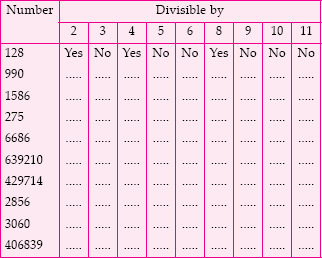SOLUTION: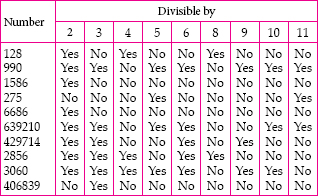Q 18.

Using divisibility tests, determine which of the following numbers are divisible by 4; by 8:
(A) 572
(B) 726352
(C) 5500
(D) 6000
(E) 12159
(F) 14560
(G) 21084
(H) 31795072
(I) 1700
(j) 2150

SOLUTION:

(A) 572 is divisible by 4 as its last two digits are divisible by 4, but it is not divisible by 8 as its last three digits are not divisible by 8.
(B) 726352 is divisible by 4 as its last two digits are divisible by 4 and it is also divisible by 8 as its last three digits are divisible by 8.
(C) 5500 is divisible by 4 as its last two digits are divisible by 4, but it is not divisible by 8 as its last three digits are not divisible by 8.
(D) 6000 is divisible by 4 as its last two digits are divisible by 4 and it is also divisible by 8 as its last three digits are divisible by 8.
(E) 12159 is not divisible by 4 and 8 as it is an odd number.
(F) 14560 is divisible by 4 as its last two digits are divisible by 4 and it is also divisible by 8 as its last three digits are divisible by 8.
(G) 21084 is divisible by 4 as its last two digits are divisible by 4, but it is not divisible by 8 as its last three digits are not divisible by 8.
(H) 31795072 is divisible by 4 as its last two digits are divisible by 4 and it is also divisible by 8 as its last three digits are divisible by 8.
(I) 1700 is divisible by 4 as its last two digits are divisible by 4, but it is not divisible by 8 as its last three digits are not divisible by 8.
(j) 2150 is not divisible by 4 as its last two digits are not divisible by 4 and it is not divisible by 8 as its last three digits are not divisible by 8.

Q 19.

Using divisibility tests, determine which of following numbers are divisible by 6:
(A) 297144
(B) 1258
(C) 4335
(D) 61233
(E) 901352
(F) 438750
(G) 1790184
(H) 12583
(I) 639210
(j) 17852

SOLUTION:

(A) 297144 is divisible by 2 as its ones place is an even number and it is also divisible by 3 as sum of its digits (= 27) is divisible by 3.
Since, the number is divisible by both 2 and 3. Therefore, it is also divisible by 6.
(B) 1258 is divisible by 2 as its ones place is an even number, but it is not divisible by 3 as sum of its digits (= 16) is not divisible by 3.
Since, the number is not divisible by both 2 and 3. Therefore, it is not divisible by 6.
(C) 4335 is not divisible by 2 as its ones place is not an even number, but it is divisible by 3 as sum of its digits (= 15) is divisible by 3.
Since, the number is not divisible by both 2 and 3. Therefore, it is not divisible by 6.
(D) 61233 is not divisible by 2 as its ones place is not an even number, but it is divisible by 3 as sum of its digits (= 15) is divisible by 3.
Since, the number is not divisible by both 2 and 3. Therefore, it is not divisible by 6.
(E) 901352 is divisible by 2 as its ones place is an even number, but it is not divisible by 3 as sum of its digits (= 20) is not divisible by 3.
Since, the number is not divisible by both 2 and 3. Therefore, it is not divisible by 6.
(F) 438750 is divisible by 2 as its ones place is an even number and it is also divisible by 3 as sum of its digits (= 27) is divisible by 3.
Since, the number is divisible by both 2 and 3. Therefore, it is also divisible by 6.
(G) 1790184 is divisible by 2 as its ones place is an even number and it is also divisible by 3 as sum of its digits (= 30) is divisible by 3.
Since, the number is divisible by both 2 and 3. Therefore, it is also divisible by 6.
(H) 12583 is not divisible by 2 as its ones place is not an even number and it is also not divisible by 3 as sum of its digits (= 19) is not divisible by 3.
Since, the number is not divisible by both 2 and 3. Therefore, it is not divisible by 6.
(I) 639210 is divisible by 2 as its ones place is an even number and it is also divisible by 3 as sum of its digits (= 21) is divisible by 3.
Since, the number is divisible by both 2 and 3. Therefore, it is divisible by 6.
(j) 17852 is divisible by 2 as its ones place is an even number, but it is not divisible by 3 as sum of its digits (= 23) is not divisible by 3.
Since, the number is not divisible by both 2 and 3. Therefore, it is not divisible by 6.

Q 20.

Using divisibility tests, determine which of the following numbers are divisible by 11:
(A) 5445
(B) 10824
(C) 7138965
(D) 70169308
(E) 10000001
(F) 901153

SOLUTION:

(A) In 5445, sum of the digits at odd places = 5 + 4 = 9
Sum of the digits at even places = 4 + 5 = 9
Difference of both sums = 9 - 9 = 0
Since the difference is 0. Therefore, the number is divisible by 11.
(B) In 10824, sum of the digits at odd places
= 4 + 8 + 1 = 13
Sum of the digits at even places = 2 + 0 = 2
Difference of both sums = 13 - 2 = 11
Since the difference is divisible by 11. Therefore, the number is divisible by 11.
(C) In 7138965, sum of the digits at odd places
= 5 + 9 + 3 + 7 = 24
Sum of the digits at even places = 6 + 8 + 1 = 15
Difference of both sums = 24 - 15 = 9
Since the difference is neither 0 nor divisible by 11. Therefore, the number is not divisible by 11.
(D) In 70169308, sum of the digits at odd places = 8 + 3 + 6 + 0 = 17
Sum of the digits at even places = 0 + 9 + 1 + 7 = 17
Difference of both sums = 17 - 17 = 0
Since the difference is 0. Therefore, the number is divisible by 11.
(E) In 10000001, sum of the digits at odd places = 1 + 0 + 0 + 0 = 1
Sum of the digits at even places
= 0 + 0 + 0 + 1 = 1
Difference of both sums = 1 - 1 = 0
Since the difference is 0. Therefore, the number is divisible by 11.
(F) In 901153, sum of the digits at odd places
= 3 + 1 + 0 = 4
Sum of the digits at even places = 5 + 1 + 9 = 15
Difference of both sums = 15 - 4 = 11
Since the difference is 11. Therefore, the number is divisible by 11.

Q 21.

Write the smallest digit and the greatest digit in the blank space of each of the following numbers so that the number formed is divisible by 3 :
(A) __ 6724
(B) 4765 __ 2

SOLUTION:

We know that a number is divisible by 3 if the sum of all digits is divisible by 3.
(A) The smallest digit will be 2.
∴ The number formed is 26724 and
2 + 6 + 7 + 2 + 4 = 21, which is divisible by 3.
And the greatest digit will be 8.
∴ The number formed is 86724 and
8 + 6 + 7 + 2 + 4 = 27, which is divisible by 3.
(B) The smallest digit will be 0.
∴ The number formed is 476502 and
4 + 7 + 6 + 5 + 0 + 2 = 24, which is divisible by 3.
And the greatest digit will be 9.
∴ The number formed is 476592 and
4 + 7 + 6 + 5 + 9 + 2 = 33, which is divisible by 3.

Q 22.

Write a digit in the blank space of each of the following numbers so that the number formed is divisible by 11 :
(A) 92 __ 389
(B) 8 __ 9484

SOLUTION:

We know that a number is divisible by 11 if the difference of the sum of the digits at odd places and that of even places is either 0 or divisible by 11.
(A) The number formed is 928389.
sum of digits at odd places = 9 + 3 + 2 = 14
sum of digits at even places = 8 + 8 + 9 = 25
Their difference = 25 - 14 = 11, which is divisible by 11.
(B) The number formed is 869484.
sum of digits at odd places = 4 + 4 + 6 = 14
sum of digits at even places = 8 + 9 + 8 = 25
Their difference = 25 - 14 = 11, which is divisible by 11.

Q 23.

Find the common factors of :
(A) 20 and 28
(B) 15 and 25
(C) 35 and 50
(D) 56 and 120

SOLUTION:

(A) Factors of 20 are 1, 2, 4, 5, 10 and 20.
Factors of 28 are 1, 2, 4, 7, 14 and 28.
∴ Common factors of 20 and 28 are 1, 2 and 4.
(B) Factors of 15 are 1, 3, 5 and 15.
Factors of 25 are 1, 5 and 25.
∴ Common factors of 15 and 25 are 1 and 5.
(C) Factors of 35 are 1, 5, 7 and 35.
Factors of 50 are 1, 2, 5, 10, 25 and 50.
∴ Common factors of 35 and 50 are 1 and 5.
(D) Factors of 56 are 1, 2, 4, 7, 8, 14, 28 and 56.
Factors of 120 are 1, 2, 3, 4, 5, 6, 8, 10, 12, 15, 20, 24, 30, 40, 60 and 120.
∴ Common factors of 56 and 120 are 1, 2, 4 and 8.

Q 24.

Find the common factors of :
(A) 4, 8 and 12
(B) 5, 15 and 25

SOLUTION:

(A) Factors of 4 are 1, 2 and 4.
Factors of 8 are 1, 2, 4 and 8.
Factors of 12 are 1, 2, 3, 4, 6 and 12.
∴ Common factors of 4, 8 and 12 are 1, 2 and 4.
(B) Factors of 5 are 1 and 5.
Factors of 15 are 1, 3, 5 and 15.
Factors of 25 are 1, 5 and 25.
∴ Common factors of 5, 15 and 25 are 1 and 5.

Q 25.

Find first three common multiples of :
(A) 6 and 8
(B) 12 and 18

SOLUTION:

(A) Multiples of 6 are 6, 12, 18, 24, 30, 36, 42, 48, 54, 60, 66, 72, ..........
Multiples of 8 are 8, 16, 24, 32, 40, 48, 56, 64, 72, ..........
∴ First three common multiples of 6 and
8 are 24, 48 and 72.
(B) Multiples of 12 are 12, 24, 36, 48, 60, 72, 84, 96, 108, 120, ..........
Multiples of 18 are 18, 36, 54, 72, 90, 108, 126, ..........
∴ First three common multiples of 12 and 18 are 36, 72 and 108.

Q 26.

Write all the numbers less than 100 which are common multiples of 3 and 4.

SOLUTION:

Multiples of 3 are 3, 6, 9, 12, 15, 18, 21, 24, 27, 30, 33, 36, 39, 42, 45, 48, 51, 54, 57, 60, 63, 66, 69, 72, 75, 78, 81, 84, 87, 90, 93, 96, 99, .......
Multiples of 4 are 4, 8, 12, 16, 20, 24, 28, 32, 36, 40, 44, 48, 52, 56, 60, 64, 68, 72, 76, 80, 84, 88, 92, 96, 100, .......
∴ Common multiples of 3 and 4 which are less than 100 are 12, 24, 36, 48, 60, 72, 84 and 96.

Q 27.

Which of the following numbers are co-prime?
(A) 18 and 35
(B) 15 and 37
(C) 30 and 415
(D) 17 and 68
(E) 216 and 215
(F) 81 and 16

SOLUTION:

(A) Factors of 18 are 1, 2, 3, 6, 9 and 18.
Factors of 35 are 1, 5, 7 and 35.
Common factor of 18 and 35 is 1.
Since, both have only one common factor, i.e., 1. Therefore, 18 and 35 are co-prime numbers.
(B) Factors of 15 are 1, 3, 5 and 15.
Factors of 37 are 1 and 37.
Common factor of 15 and 37 is 1.
Since, both have only one common factor, i.e., 1. Therefore, 15 and 37 are co-prime numbers.
(C) Factors of 30 are 1, 2, 3, 5, 6, 10, 15 and 30.
Factors of 415 are 1, 5, ............, 83 and 415.
Common factors of 30 and 415 are 1 and 5.
Since, both have more than one common factor. Therefore, 30 and 415 are not co-prime numbers.
(D) Factors of 17 are 1 and 17.
Factors of 68 are 1, 2, 4, 17, 34 and 68.
Common factors of 17 and 68 are 1 and 17.
Since, both have more than one common factor. Therefore, 17 and 68 are not co-prime numbers.
(E) Factors of 216 are 1, 2, 3, 4, 6, 8, 9, 12, 18, 24, 27, 36, 54, 72, 108 and 216.
Factors of 215 are 1, 5, 43 and 215.
Common factor of 216 and 215 is 1.
Since, both have only one common factor, i.e., 1. Therefore, 216 and 215 are co-prime numbers.
(F) Factors of 81 are 1, 3, 9, 27 and 81.
Factors of 16 are 1, 2, 4, 8 and 16.
Common factor of 81 and 16 is 1.
Since, both have only one common factor, i.e., 1. Therefore, 81 and 16 are co-prime numbers.

Q 28.

A number is divisible by both 5 and 12. By which other number will that number be always divisible?

SOLUTION:

Since 5 × 12 = 60. The number divisible by both 5 and 12, must also be divisible by 60.

Q 29.

A number is divisible by 12. By what other numbers will that number be divisible?

SOLUTION:

Factors of 12 are 1, 2, 3, 4, 6 and 12.
Therefore, the number divisible by 12, will also be divisible by 1, 2, 3, 4 and 6.

Q 30.

Which of the following statements are true?
(A) If a number is divisible by 3, it must be divisible by 9.
(B) If a number is divisible by 9, it must be divisible by 3.
(C) A number is divisible by 18, if it is divisible by both 3 and 6.
(D) If a number is divisible by 9 and 10 both, then it must be divisible by 90.
(E) If two numbers are co-primes, at least one of them must be prime.
(F) All numbers which are divisible by 4 must also be divisible by 8.
(G) All numbers which are divisible by 8 must also be divisible by 4.
(H) If a number exactly divides two numbers separately, it must exactly divide their sum.
(I) If a number exactly divides the sum of two numbers, it must exactly divide the two numbers separately.

SOLUTION:

Statements (B), (D), (G) and (H) are true.

Q 31.

Here are two different factor trees for 60. Write the missing numbers.
(A)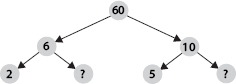(B)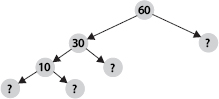SOLUTION:

Factors of 60 are 1, 2, 3, 4, 5, 6, 10, 12, 15, 20, 30, 60.
(A) Since 6 = 2 × 3 and 10 = 5 × 2
∴ The missing numbers are 3 and 2.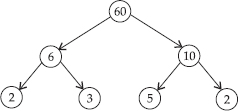(B) Since, 60 = 30 × 2, 30 = 10 × 3 and 10 = 5 × 2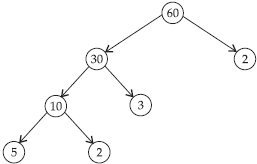Q 32.

Which factors are not included in the prime factorisation of a composite number?

SOLUTION:

1 and the number itself are not included in the prime factorisation of a composite number.

Q 33.

Write the greatest 4-digit number and express it in terms of its prime factors.

SOLUTION:

The greatest four digit number is 9999.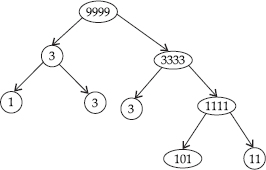∴ 9999 = 3 × 3 × 11 × 101.

Q 34.

Write the smallest 5-digit number and express it in the form of its prime factors.

SOLUTION:

The smallest five digit number is 10000.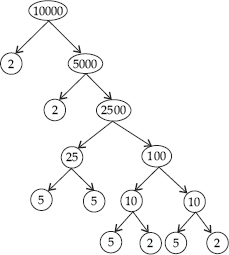∴ 10000 = 2 × 2 × 2 × 2 × 5 × 5 × 5 × 5.

Q 35.

Find all the prime factors of 1729 and arrange them in ascending order. Now state the relation, if any; between two consecutive prime factors.

SOLUTION: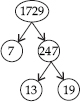∴ 1729 = 7 × 13 × 19.
The difference of two consecutive prime factors is 6. (∵ 13 - 7 = 6 and 19 - 13 = 6)

Q 36.

The product of three consecutive numbers is always divisible by 6. Verify this statement with the help of some examples.

SOLUTION:

Among the three consecutive numbers, there must be atleast one even number and one multiple of 3. Thus, the product must be divisible by 6.
For example : (I) 2 × 3 × 4 = 24
(ii) 4 × 5 × 6 = 120,
where both 24 and 120 are divisible by 6.

Q 37.

The sum of two consecutive odd numbers is divisible by 4. Verify this statement with the help of some examples.

SOLUTION:

The sum of two consecutive odd numbers is divisible by 4.
For example : 3 + 5 = 8 and 8 is divisible by 4.
5 + 7 = 12 and 12 is divisible by 4.
7 + 9 = 16 and 16 is divisible by 4.
9 + 11 = 20 and 20 is divisible by 4.

Q 38.

In which of the following expressions, prime factorisation has been done?
(A) 24 = 2 × 3 × 4
(B) 56 = 7 × 2 × 2 × 2
(C) 70 = 2 × 5 × 7
(D) 54 = 2 × 3 × 9

SOLUTION:

In expressions (B) and (C), prime factorisation has been done.

Q 39.

Determine if 25110 is divisible by 45.
[Hint : 5 and 9 are co-prime numbers. Test the divisibility of the number by 5 and 9].

SOLUTION:

The prime factorisation of 45 = 5 × 9
25110 is divisible by 5 as '0' is at its unit place.
25110 is divisible by 9 as sum of digits (i.e., 9) is divisible by 9.
Therefore, the number 25110 must be divisible by 5 × 9 = 45.

Q 40.

18 is divisible by both 2 and 3. It is also divisible by 2 × 3 = 6. Similarly, a number is divisible by both 4 and 6. Can we say that the number must also be divisible by 4 × 6 = 24? If not, give an example to justify your answer.

SOLUTION:

No. The number 12 is divisible by both 6 and 4, but 12 is not divisible by 24.
∴ A number divisible by both 4 and 6 may or may not be divisible by 4 × 6 = 24.

Q 41.

I am the smallest number, having four different prime factors. Can you find me?

SOLUTION:

Since, 2 × 3 × 5 × 7 = 210
∴ 210 is the smallest number, having 4 different prime factors i.e., 2, 3, 5 and 7.

Q 42.

Find the HCF of the following numbers :
(A) 18, 48
(B) 30, 42
(C) 18, 60
(D) 27, 63
(E) 36, 84
(F) 34, 102
(G) 70, 105, 175
(H) 91, 112, 49
(I) 18, 54, 81
(j) 12, 45, 75

SOLUTION:

(A) The prime factorisation of 18 and 48 are; 18 = 2 × 3 × 3
48 = 2 × 2 × 2 × 2 × 3
∴ HCF (18, 48) = 2 × 3 = 6
(B) The prime factorisation of 30 and 42 are;
30 = 2 × 3 × 5
42 = 2 × 3 × 7
∴ HCF (30, 42) = 2 × 3 = 6
(C) The prime factorisation of 18 and 60 are;
18 = 2 × 3 × 3
60 = 2 × 2 × 3 × 5
∴ HCF (18, 60) = 2 × 3 = 6
(D) The prime factorisation of 27 and 63 are;
27 = 3 × 3 × 3
63 = 3 × 3 × 7
∴ HCF (27, 63) = 3 × 3 = 9
(E) The prime factorisation of 36 and 84 are;
36 = 2 × 2 × 3 × 3
84 = 2 × 2 × 3 × 7
∴ HCF (36, 84) = 2 × 2 × 3 = 12
(F) The prime factorisation of 34 and 102 are;
34 = 2 × 17
102 = 2 × 3 × 17
∴ HCF (34, 102) = 2 × 17 = 34
(G) The prime factorisation of 70, 105 and 175 are; 70 = 2 × 5 × 7
105 = 3 × 5 × 7
175 = 5 × 5 × 7
∴ HCF (70, 105, 175) = 5 × 7 = 35
(H) The prime factorisation of 91, 112 and 49 are;
91 = 7 × 13
112 = 2 × 2 × 2 × 2 × 7
49 = 7 × 7
∴ HCF (91, 112, 49) = 7
(I) The prime factorisation of 18, 54 and 81 are; 18 = 2 × 3 × 3
54 = 2 × 3 × 3 × 3
81 = 3 × 3 × 3 × 3
∴ HCF (18, 54, 81) = 3 × 3 = 9
(j) The prime factorisation of 12, 45 and 75 are; 12 = 2 × 2 × 3
45 = 3 × 3 × 5
75 = 3 × 5 × 5
∴ HCF (12, 45, 75) = 3

Q 43.

What is the HCF of two consecutive
(A) numbers?
(B) even numbers?
(C) odd numbers?

SOLUTION:

(A) HCF of two consecutive numbers is 1.
(B) HCF of two consecutive even numbers is 2.
(C) HCF of two consecutive odd numbers is 1.

Q 44.

HCF of co-prime numbers 4 and 15 was found as follows by factorisation :
4 = 2 × 2 and 15 = 3 × 5 since there is no common prime factor, so HCF of 4 and 15 is 0. Is the answer correct? If not, what is the correct HCF?

SOLUTION:

No. The correct HCF of 4 and 15 is 1.

Q 45.

Renu purchases two bags of fertiliser of weights 75 kg and 69 kg. Find the maximum value of weight which can measure the weight of the fertiliser exact number of times.

SOLUTION:

For finding maximum weight, we have to find HCF of 75 and 69.
The prime factorisation of 75 and 69 are;
75 = 3 × 5 × 5, 69 = 3 × 23
So, HCF of 75 and 69 = 3
Therefore, the required weight is 3 kg.

Q 46.

Three boys step off together from the same spot. Their steps measure 63 cm, 70 cm and
77 cm respectively. What is the minimum distance each should cover so that all can cover the distance in complete steps?

SOLUTION:

For finding minimum distance, we have to find LCM of 63, 70, 77.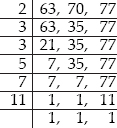LCM of 63, 70 and 77 = 2 × 3 × 3 × 5 × 7 × 11 = 6930
Therefore, the minimum distance three boys should cover is 6930 cm.

Q 47.

The length, breadth and height of a room are 825 cm, 675 cm and 450 cm respectively.
Find the longest tape which can measure the three dimensions of the room exactly.

SOLUTION:

The measurement of the longest tape
= HCF of 825 cm, 675 cm and 450 cm.
The prime factorisation of 825, 675 and 450 are;
825 = 3 × 5 × 5 × 11
675 = 3 × 3 × 3 × 5 × 5
450 = 2 × 3 × 3 × 5 × 5
Now, HCF of 825, 675 and 450 = 3 × 5 × 5 = 75
Therefore, the measurement of longest tape is 75 cm.

Q 48.

Determine the smallest 3-digit number which is exactly divisible by 6, 8 and 12.

SOLUTION:

The smallest 3-digit number = 100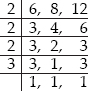LCM of 6, 8 and 12 = 2 × 2 × 2 × 3 = 24
To find the number, we have to divide 100 by 24.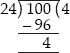Therefore, the required number = 100 + (24 - 4) = 120.

Q 49.

Determine the greatest 3-digit number exactly divisible by 8, 10 and 12.

SOLUTION:

The greatest three digit number = 999.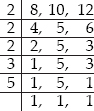LCM of 8, 10 and 12 = 2 × 2 × 2 × 3 × 5 = 120
Now, to find the number, we have to divide 999 by 120.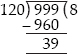Therefore, the required number = 999 - 39 = 960

Q 50.

The traffic lights at three different road crossings change after every 48 seconds, 72 seconds and 108 seconds respectively. If they change simultaneously at 7 a.m., at what time will they change simultaneously again?

SOLUTION: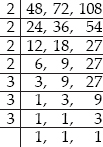LCM of 48, 72 and 108 = 2 × 2 × 2 × 2 × 3 × 3 × 3 = 432
After 432 seconds, the traffic lights change simultaneously.
432 seconds = 7 minutes 12 seconds
Therefore, the required time = 7 a.m. + 7 minutes 12 seconds i.e., 7 minutes 12 seconds past 7 a.m.

Q 51.

Three tankers contain 403 litres, 434 litres and 465 litres of diesel respectively. Find the maximum capacity of a container that can measure the diesel of the three containers exact number of times.

SOLUTION:

The maximum capacity of a container
= HCF (403, 434, 465)
The prime factorisation of 403, 434 and 465 are;
403 = 13 × 31
434 = 2 × 7 × 31
465 = 3 × 5 × 31
HCF of 403, 434 and 465 = 31
Therefore, a container of capacity 31 litres is required to measure the diesel of the three containers.

Q 52.

Find the least number which when divided by 6, 15 and 18 leave remainder 5 in each case.

SOLUTION: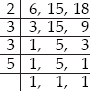LCM of 6, 15 and 18 = 2 × 3 × 3 × 5 = 90
Therefore, the required number = 90 + 5 = 95

Q 53.

Find the smallest 4-digit number which is divisible by 18, 24 and 32.

SOLUTION:

The smallest four digit number = 1000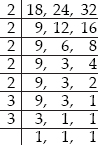LCM of 18, 24 and 32 = 2 × 2 × 2 × 2 × 2 × 3 × 3 = 288
Now,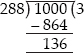Therefore, the required number is
1000 + (288 - 136) = 1152.

Q 54.

Find the LCM of the following numbers :
(A) 9 and 4
(B) 12 and 5
(C) 6 and 5
(D) 15 and 4
Observe a common property in the obtained LCMs. Is LCM the product of two numbers in each case?

SOLUTION:

(A) We have,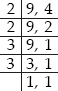LCM of 9 and 4 = 2 × 2 × 3 × 3 = 36
(B) We have,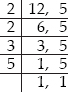LCM of 12 and 5 = 2 × 2 × 3 × 5 = 60
(C) We have,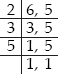LCM of 6 and 5 = 2 × 3 × 5 = 30
(D) We have,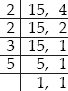LCM of 15 and 4 = 2 × 2 × 3 × 5 = 60
Yes, the LCM is equal to the product of two numbers in each case and all LCMs are also the multiple of 3.

Q 55.

Find the LCM of the following numbers in which one number is the factor of the other.
(A) 5, 20
(B) 6, 18
(C) 12, 48
(D) 9, 45
What do you observe in the results obtained?

SOLUTION:

(A) We have,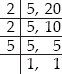LCM of 5 and 20 = 2 × 2 × 5 = 20
(B) We have,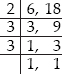LCM of 6 and 18 = 2 × 3 × 3 = 18
(C) We have,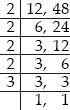LCM of 12 and 48 = 2 × 2 × 2 × 2 × 3 = 48
(D) We have,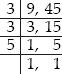LCM of 9 and 45 = 3 × 3 × 5 = 45
From above, we observe that the LCM of the given numbers in each case is the larger of the two numbers.